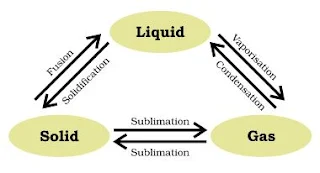#### Solutions of Science CBSE Sample Paper Class 9th Summative Assessment I 2016 SET A

1. Amoeba and Paramecium
2. Acceleration
3. Ampere
4. Cork are formed from the layers of cells when strip of secondary meristem replaces the epidermis of the stem.
5.6. Acceleration = a = 10 ms-2
Initial speed = u = 0
Time = t = 2s
Distance = s = ut + 1/2 at2 = (0×2) + (1/2 × 10 × 22 ) = 0 + 20 = 20m

7. When the state of a substance changes, then the temperature of the substance remains constant because the heat that is supplied gets used up in overcoming the force of attraction between the particles.
When solid melts on heating, its temperature does not rise until the whole solid converted into liquid because the heat supplied used up in overcoming the attraction between the particles of solid.

8. The space between the particles of gas is very large. When pressure is applied on it, the particles of gases come closer to each other which develops force of attraction and thus help in liquefaction of gases.
LPG and liquid acetylene are examples two liquefied gases.

9.The colour of the solution changes into green.
Fe + CuSO4 → FeSO4 + Cu

10. (i) Auxins
(ii) Auxins
(iii) Abscisic acid

11. Four types of epithelial tissues are:
(i) Squamous
(ii) Cuboidal
(iii) Columnar
(iv) Transitional
Epithelial tissues cover body surfaces and lines body cavities.

12. Total distance covered = 700 + 300 + 400 + 100 + 1200 + 300 + 100 = 3100 m
Displacement in north and south = 700 + 400 + 100 - 1200 = 0
Displacement in east and west = 300 + 300 - 100 = 500 m in east

13. (a) Velocity
(b) Displacement
(c) Acceleration

14. Mass will be same on planet M.
Weight on planet M = 50 × 9.8 × 3 = 1470 N

15. Newton's first law of motion: It states that an object will remain at rest or in uniform motion in a straight line unless acted upon by an external force.
Newton's second law of motion: The acceleration of an object as produced by a force is directly proportional to the magnitude of the net force in the same direction and inversely proportional to the mass of the object.
Newton's third law of motion: For every action there is always equal and opposite reaction.

16. (a) Lactation period defines as the period when cow is producing milk mostly after giving birth to calf.
(b) Jersey, Brown Swiss
(c) Vishnu exhibits the quality of scientific and logical thinking thus helping the society economically.

17. Mixed cropping is growing of two or more crops simultaneously on the same piece of land.